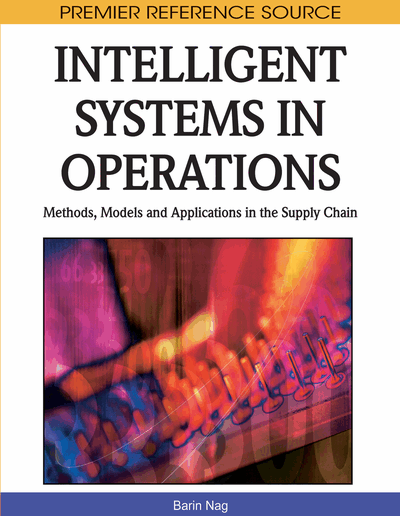# SAT and Planning: An Overview

Carlos Camarão (University of Minas Gerais, Brazil), Mateus Galvão (University of Minas Gerais, Brazil) and Newton Vieira (University of Minas Gerais, Brazil)
Copyright: © 2010 |Pages: 14
DOI: 10.4018/978-1-61520-605-6.ch002
OnDemand PDF Download:
Available
\$37.50
No Current Special Offers

## Abstract

This chapter firstly reviews the importance of the Satisfiability Problem (SAT) for a wide range of applications, including applications in Operation Management such as planning. A review of methods nowadays employed by modern SAT-solvers is then presented. The authors then use Classical Planning as an illustrative example of how a significant problem can be translated into SAT. They point out important results and studies concerning reductions of planning into SAT, and explain how to construct a SAT instance which is satisfiable if and only if an instance of a bounded version of the classic blocks-world problem is solvable.
Chapter Preview
Top

## Introduction

Twenty years ago the use of formal logic as a basis for knowledge representation and processing was subject to severe critics, founded not only on problems of expressiveness, but also on problems of running time performance. Since that time the problem of expressivity has been object of intense research, which resulted in extensions and alternative formulations of logics intended to deal with important aspects of “intelligent” systems not dealt with by classical formal logic (the logic underlying purely mathematical reasoning) (Brachman & Levesque, 2004). From the point of view of running time performance, significant advancements have also been achieved (A. Robinson & Voronkov, 2001; Harmelen, Lifschitz, & Porter, 2008), even considering the undecidability of more expressive logics.

Although knowledge expression and processing in the area of artificial intelligence is still subject to intensive research, far from providing the necessary basis for its highest objectives (as the production of intelligent behavior), the last years have seen a growing use of logic based systems for solving many industrial level applications in several domains.

In this chapter one of the simplest logics from the standpoint of expressivity will be considered: propositional logic. In spite of its simplicity, it is sufficient for the description and solution of an enormous amount of practical problems. Even considering that other logics are more adequate from a notational point of view, in order to have more concise descriptions for certain application classes, in many cases it is possible to produce automatically a translation to propositional logic (Cadoli & Schaerf, 2005; Navarro, 2007).

The justification for using a knowledge processor based on propositional logic, even in some situations where more expressive logics would be apparently more adequate (by having more concise sentences), is that the actual programs for propositional logic processing are so advanced that they allow the solution of many practical problem instances. They are typically not only more efficient than respective programs for other logics, but also than specific programs specially tailored to the application at hand (Gomes, Kautz, Sabharwal, & Selman, 2008).

The idea underlying the use of formal logic for problem solving is to obtain a solution for a problem instance from a given formal specification of the problem expressed in the language of that logic. There are two basic approaches for achieving this goal. In the approach based on proof theory, finding a solution consists of finding a proof for a formula α from a set of formulas Σ. For example, in the problems of software or hardware verification, Σ would be a formal description of an algorithm or a digital circuit and α a property to be verified. Another example: to verify the equivalence of two circuit descriptions given by two formulas α and ß it is sufficient to establish the validity of the formula α↔ß or, in other words, to prove that α ↔ ß follows from the empty set of hypotheses. A huge number of other kinds of problems can be formulated as that of obtaining a proof of a formula (theorem) α from a set of formulas (axioms or hypotheses) Σ (Larry, 1984; Lifschitz, Morgenstern, & Plaisted, 2008).

## Complete Chapter List

Search this Book:
Reset